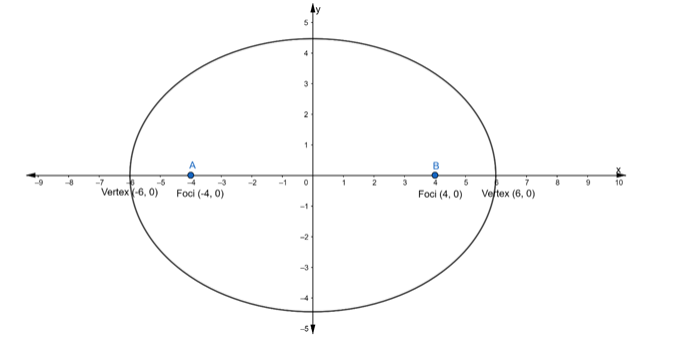# Find the equation of the ellipse whose vertices are at`
Question:

Find the equation of the ellipse whose vertices are at (±6, 0) and foci at (±4, 0).

Solution:Given: Vertices $=(\pm 6,0) \ldots$ (i)

The vertices are of the form $=(\pm a, 0) \ldots$ (ii)

Hence, the major axis is along $x$-axis

$\therefore$ From eq. (i) and (ii), we get

$a=6$

$\Rightarrow a^{2}=36$

and We know that, if the major axis is along x – axis then the equation of Ellipse is of the form of

$\frac{x^{2}}{a^{2}}+\frac{y^{2}}{b^{2}}=1$

Also, given coordinate of foci $=(\pm 4,0) \ldots$ (iii)

We know that,

Coordinates of foci $=(\pm c, 0)$...(iv)

$\therefore$ From eq. (iii) and (iv), we get

$c=4$

We know that,

$c^{2}=a^{2}-b^{2}$

$\Rightarrow(4)^{2}=(6)^{2}-b^{2}$

$\Rightarrow 16=36-b^{2}$

$\Rightarrow b^{2}=36-16$

$\Rightarrow b^{2}=20$

Substituting the value of $a^{2}$ and $b^{2}$ in the equation of an ellipse, we get

$\frac{x^{2}}{a^{2}}+\frac{y^{2}}{b^{2}}=1$

$\Rightarrow \frac{x^{2}}{36}+\frac{y^{2}}{20}=1$